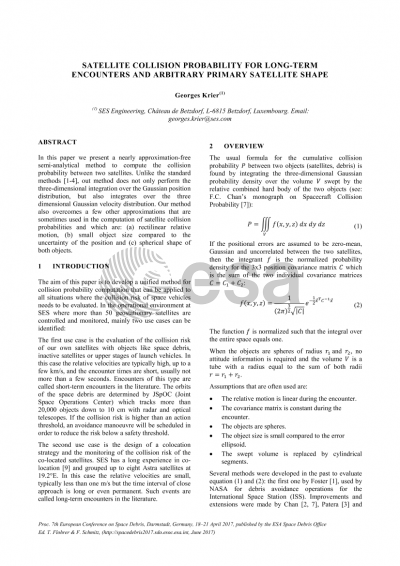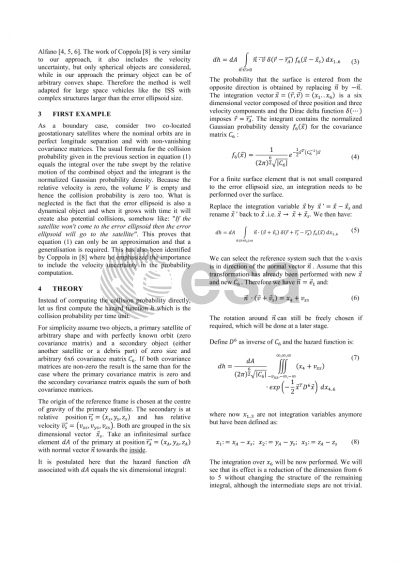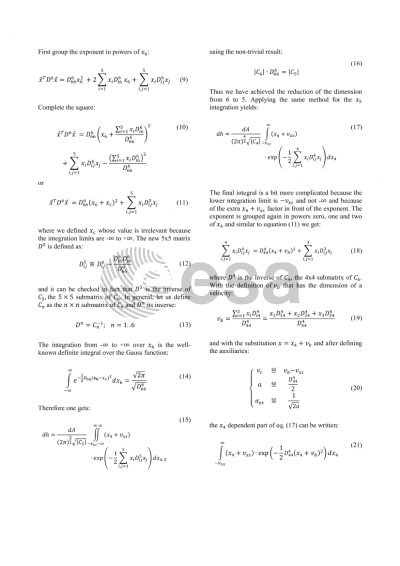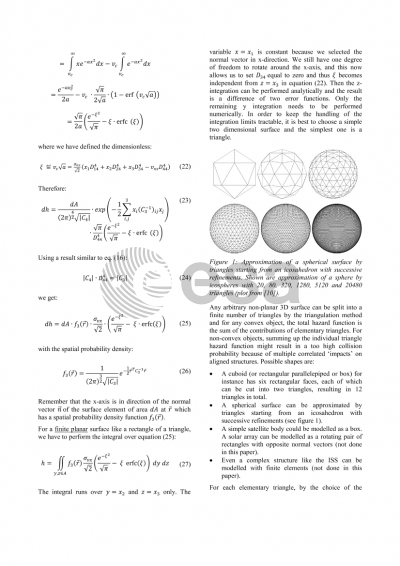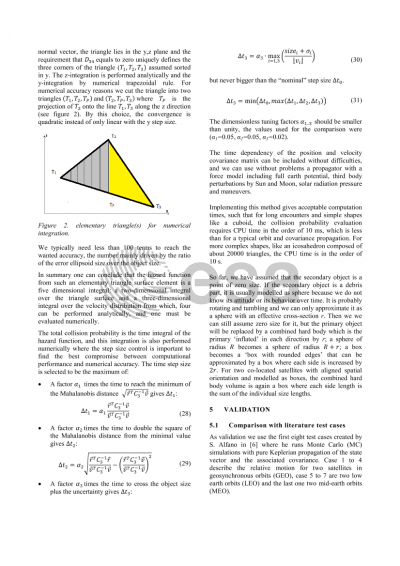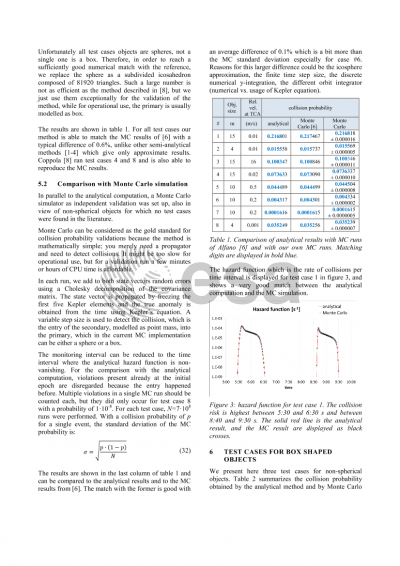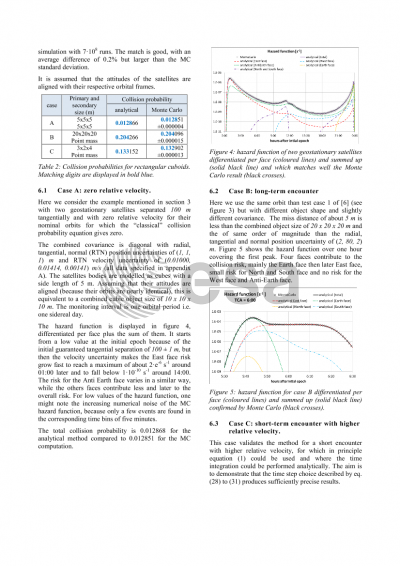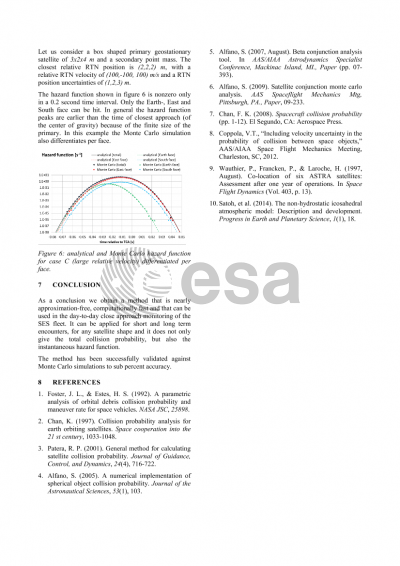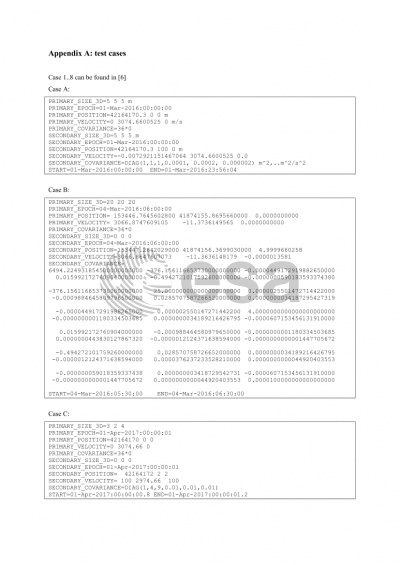# Satellite collision probability for long-term encounters and arbitrary primary satellite shape.

Georges Krier1
SES Engineering1

## Document details

Publishing year2017 PublisherESA Space Debris Office Publishing typeConference Name of conference7th European Conference on Space Debris
Pagesn/a Volume
7
Issue
1
Editors
T. Flohrer, F. Schmitz
Download paper## Abstract

In this paper we present a nearly approximation-free semi-analytical method to compute the collision probability between two satellites. Unlike the standard method, that evaluates the integral of the normalized Gaussian probability density over the tube swept by the relative motion of the combined object, out method does not only perform the three-dimensional integration over the Gaussian position distribution, but also integrates over the three dimensional Gaussian velocity distribution. As a boundary case, consider two co-located geostationary satellites in perfect longitude separation. The standard method obtains a collision probability of zero because the relative velocity is zero. But even if the centers of the error ellipsoids do not move, they increase with time, thus creating a larger overlap and hence a non-zero collision probability.

Our method also overcomes a few other approximations that are often used in the computation of satellite collision probabilities: (a) rectilinear relative motion, (b) small object size compared to the uncertainty of the position and (c) spherical shape of both objects.
The assumption of a spherical shape of the secondary object is not a strong limitation, because its attitude is usually unknown and it is therefore modeled as a sphere with a cross section equal to its effective cross section. In order to overcome the approximation of the spherical shape of the primary object, its surface is subdivided into small triangles, whose size is determined by the object shape and the error ellipsoid size. Each triangle contributes additively to the hazard function (instantaneous collision probability). The hazard function from such an elementary triangle surface element is a five dimensional integral (over the two-dimensional triangle surface and the three-dimensional velocity distribution) from which, four can be performed analytically, and only the last one must be evaluated numerically. To obtain the total collision probability, the hazard function needs to be integrated in time, and this integration is also performed numerically where the step size control is important to find the best compromise between computational performance and numerical accuracy.

Note that the proposed method assumes that the swept volume can be replaced by cylindrical segments. In most practical cases, this is fulfilled because the curvature radius of the relative orbit is large compared to the combined object size.

In a second section we present the results of a Monte Carlo simulation in order validate the analytical computations. The test cases, that are taken from the literature use spherical primaries which are modeled as a subdivided icosahedron, composed by up to 20480 triangles.

As a conclusion we obtain a method that is nearly approximation-free, computationally fast and that can be used in the day-to-day close approach monitoring of the SES fleet.

## Preview